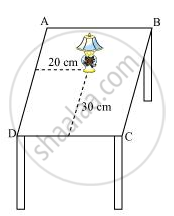# How Will You Describe the Position of a Table Lamp on Your Study Table to Another Person? - Mathematics

How will you describe the position of a table lamp on your study table to another person?

#### Solution 1Consider that the lamp is placed on the table. Choose two adjacent edges, DC and AD. Then, draw perpendiculars on the edges DC and AD from the position of lamp and measure the lengths of these perpendiculars. Let the length of these perpendiculars be 30 cm and 20 cm respectively. Now, the position of the lamp from the left edge (AD) is 20 cm and from the lower edge (DC) is 30 cm. This can also be written as (20, 30), where 20 represents the perpendicular distance of the lamp from edge AD and 30 represents the perpendicular distance of the lamp from edge DC.

#### Solution 2

For describing the position of a mobile phone kept on the study table, we take two lines, a perpendicular and a horizontal line.

Considering the table as a plane(x and y axis) and taking perpendicular line as Y axis and horizontal as X axis respectively. Take one corner of table as origin where both X and Y axes intersect each other. Now, the length of table is Y axis and breadth is X axis. From The origin, join the line to the mobile phone and mark a point. The distances of the point from both X and Y axes should be calculated and then should be written in terms of coordinates.

Let the distance of the point from X- axis and Y- axis is x and y respectively, so the mobile phone will be in (x, y) coordinate.

Concept: Coordinate Geometry
Is there an error in this question or solution?

#### APPEARS IN

NCERT Class 9 Maths
Chapter 3 Coordinate Geometry
Exercise 3.1 | Q 1 | Page 53